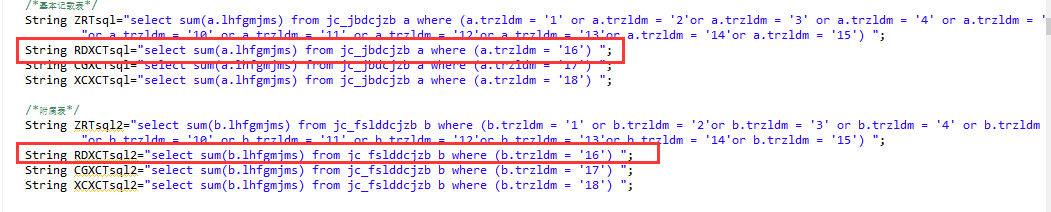# 两条求和sql语句相加...全文
580 点赞 收藏 4

4 条回复

``````select sum(n) from (
select sum(a.lhfgmjms) as n from jc_jbdcjzb a where ( a.trzldm='16' )
union all
select sum(b.lhfgmjms) as n from jc_fslddcjzb b where ( b.trzldm='16' )
) as t
``````

[quote=引用 1 楼 yenange 的回复:]
``````select sum(n) from (
select sum(a.lhfgmjms) as n from jc_jbdcjzb a where ( a.trzldm='16' )
union all
select sum(b.lhfgmjms) as n from jc_fslddcjzb b where ( b.trzldm='16' )
) as t
``````

``````select sum(n) from (
select sum(a.lhfgmjms) as n from jc_jbdcjzb a where ( a.trzldm='16' )
union all
select sum(b.lhfgmjms) as n from jc_fslddcjzb b where ( b.trzldm='16' )
) as t
``````

``````select sum(n) from (
select sum(a.lhfgmjms) as n from jc_jbdcjzb a where ( a.trzldm='16' )
union all
select sum(b.lhfgmjms) as n from jc_fslddcjzb b where ( b.trzldm='16' )
) as t
``````

2.1w+

MS-SQL Server 疑难问题